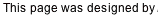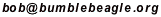The goal in a Numerichain puzzle is to uncover the location of every number in a grid of squares. In this example we have five rows and five columns, so the solution will consist of the numbers 1 through 25. The numbers will form “a chain”— 1 will be next to 2, 2 will be next to 3, and so on (diagonals don’t count).

(Below, left) Here’s what our 5x5 example looks like a the start. We have 25 squares (or “boxes”)— the numbers 1 through 25 will go in these. There are 16 circles (or “dots”) where queries can be made. Three queries have been made for us— these dots are blue. The four boxes around each blue dot are shown in light blue. This indicates that we have some information about those boxes.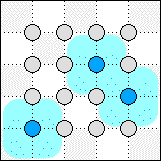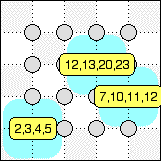(Above, right) To solve the puzzle, we’ll need to see what the queries are telling us about the surrounding boxes. Wave the cursor over each blue dot, bringing up the popup lists for the queries. For example, “2,3,4,5” tells us that the four boxes around that query contain 2, 3, 4, and 5. The tricky part is what don’t know the order; we don ’t know which box 5 goes into, which 3 goes into, and so on.

We could make additional queries by clicking on one of the gray dots. For this puzzle it’s not necessary. With a little logic we can figure out the entire number chain from just these three queries.

(Below, left) Observe that one box is common to two queries. Since 12 appears in the list for both of them, we know that 12 must go in that box.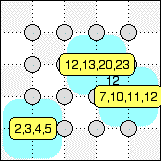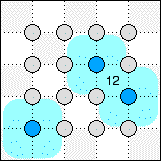(Above, right) Sometimes the popups make it hard to see what we’ve got in the grid. Clear the popups by clicking above or below the puzzle grid.

(Below, left) Bring the popups back up (by waving the cursor over them), and consider 5 and 7. These can only be two boxes apart, and the queries they are in only have two boxes that fit the bill. So we can place 5 and 7, and 6 as well. Notice that the applet adds a brown link between the numbers.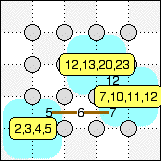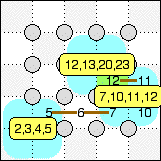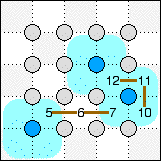(Above, middle) Now think about where 11 can go. We have already used up two of the four boxes in the 7,10,11,12 query. 11 has to be in one of the other two boxes, to the right of either 12 or 7. But it has to be next to 12 (remember, diagonals don’t count). And then 10 has to go in the fourth box.

(Above, right) Remove the popups to see what we’ve got so far.

(Below, left) We have two pieces of the chain, and eventually we’ll have to connect them. In particular, 7 will have to connect to 10 through an 8 and a 9. Think about where 8 can go. It doesn’t appear in any query. But it has to get next to 7, and there’s only one empty box next to 7. So 8 has to go there. Ditto for 9 next to 10.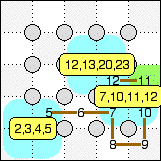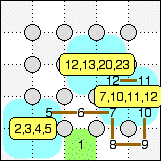(Above, right) Now think about the box below 6. What number can occupy that box? The boxes above (6) and to the right (8) are already taken. There’s no box below it. The only empty box next to it is to the left. So whatever number goes in this box, it can only link to one other number. But every number in our chain will link to two numbers, except for the ends of the chain, 1 and 25. So this box must be a 1 or a 25. It can’t be 25, since it couldn’t connect to 23. So it must be 1.

(Below, left) Now that we know where 1 is, we know where 2 must go. And 3 and 4.

(Below, middle) Here’s what we’ve got; we’re nearly halfway done.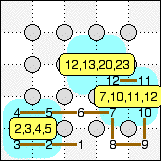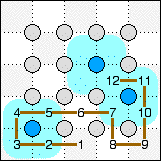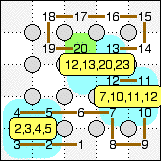(Above, right) We dont’t have a lot of information left. We know 13 has to go next to 12, either above it or to the left. Let’s try putting it above 12 and see if that will work. If we put it there, 14 will have to be to the right (this takes a little thought to see, but if 14 were above 13, we’d have trouble filling the two boxes to the right of 13 and 14). Then 15, 16, 17, and 18 must follow around the edge. And since 20 is listed in the query, 19 must come down from 18 so that it can be next to 20. But then there’s no place for 21 next to 20 (because 23 takes up the last box in the query).

(Below, left) We’ve proved 13 can’t go above 12, so it must go to the left of it.

(Below, middle) 14 has to go next to 13, and there’s only one place for it.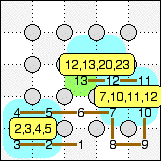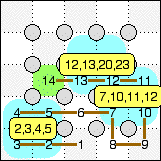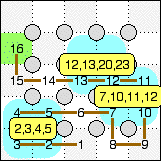(Above, right) The box to the left of 14 has to be filled. If it doesn’t connect to 14 (in other words, if it’s not 15), it could only link to one number, and would have to be 25 (since we've used the other end of the chain, 1, already). But it’s too far from 23 to be the 25. So, it must be 15. And then 16 must be above it.

(Below, left) We have a similar situation with the box above 16. We could try putting 23 as close as we can to it, then seeing how far we can stretch 25. It won’ reach.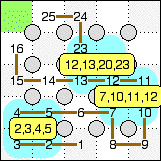(Above, right) So 17 has to go above 16. And 18 has to go to the right. Notice how we’ve been able to fill in a good part of the upper left of the puzzle, even though we have no queries over there.

(Below, left) 20 will have to be in the upper left blue box to be close enough to 18. There are two choice for where 19 can go. But if we try it above 20, the box left of 20 can never be filled.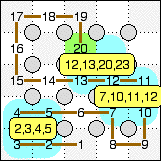(Above, right) So 19 must be left of 20.

(Below, left) That leaves only one place in the query for 23.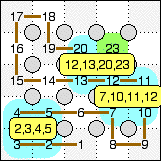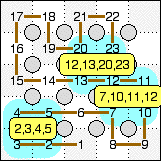(Above, right) Which forces 21 and 22 (similar to the way 8 and 9 were forced).

(Below, left) Which forces 24 and 25.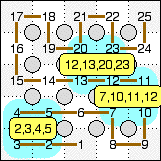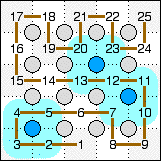(Above, right) The puzzle is now completely solved.

Back to the main Numerichain page